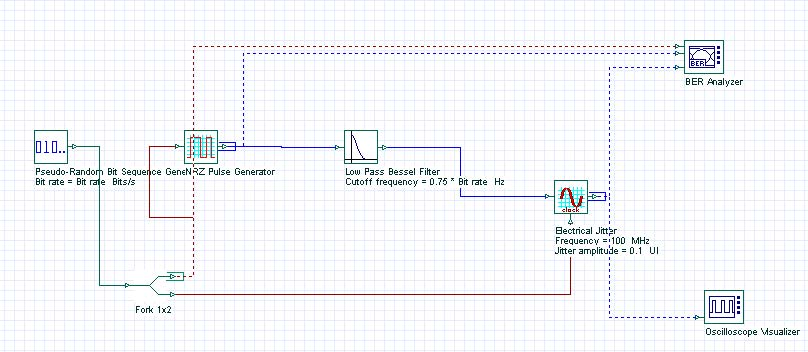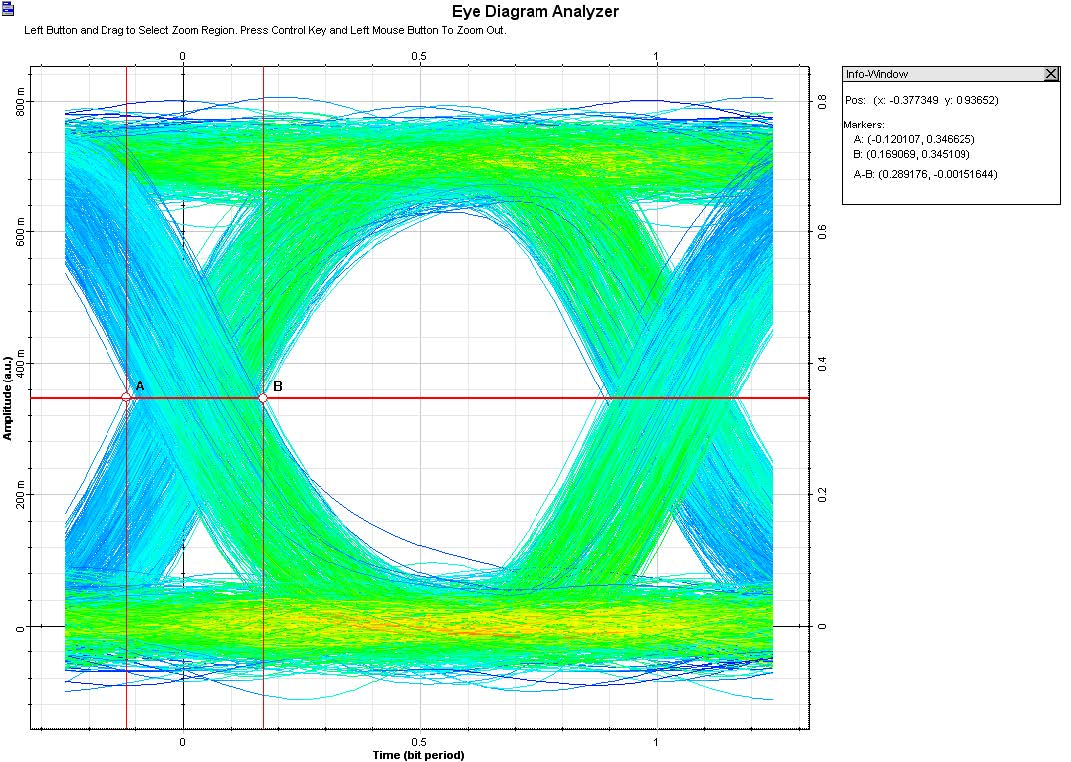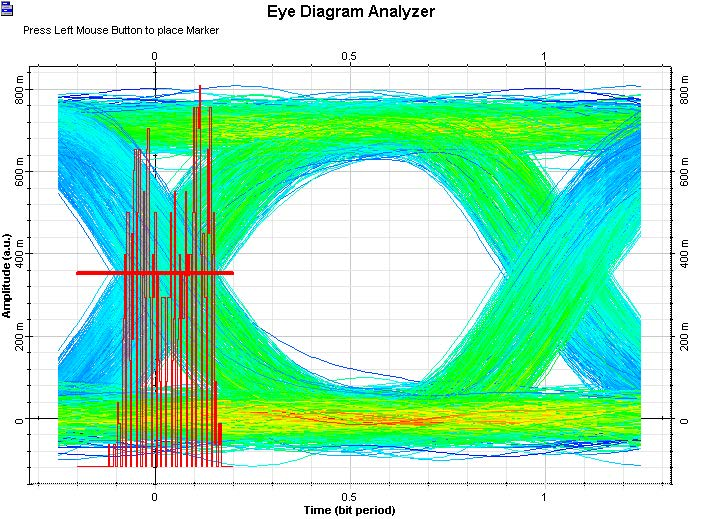Jitter is defined as the short-term variations of a digital signal’s significant instants from their ideal positions in time. Significant instants could be (for example) the optimum sampling instants. Project Signal Degradation – Jitter.osd (Figure 1) demonstrates the setup for the ‘Electrical Jitter’ component. It requires an electrical signals and the clock signal from the PRBS in order to estimate the signal bit rate. Parameter sweeps are use to generate multiple eye diagrams for different values of jitter amplitude and frequency.Figure 1: Signal degradation from jitter

Project Measuring Jitter.osd demonstrates how to measure Jitter using the Eye Diagram Analyzer histogram feature. Figure 2 shows the total jitter of an eye diagram, measured at the eye cross point, as the difference between the time values of marks A and B. Jitter is composed of two basic types: random and deterministic. In OptiSystem we can use the Eye Diagram Analyzer histogram feature to generate a histogram that shows the deterministic and random components of the jitter, as depicted in Figure 3.Figure 2: Total jitter from the eye diagramFigure 3: Using histograms to measure jitter